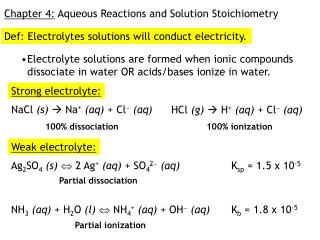DownloadDownload PresentationChapter 4: Aqueous Reactions and Solution Stoichiometry

# Chapter 4: Aqueous Reactions and Solution Stoichiometry

Télécharger la présentation## Chapter 4: Aqueous Reactions and Solution Stoichiometry

- - - - - - - - - - - - - - - - - - - - - - - - - - - E N D - - - - - - - - - - - - - - - - - - - - - - - - - - -
##### Presentation Transcript

1. Ag2SO4(s) 2 Ag+(aq) + SO42(aq) Partial dissociation NH3(aq) + H2O (l) NH4+(aq) + OH(aq) Partial ionization Chapter 4: Aqueous Reactions and Solution Stoichiometry Def: Electrolytes solutions will conduct electricity. • Electrolyte solutions are formed when ionic compounds dissociate in water OR acids/bases ionize in water. Strong electrolyte: NaCl (s) Na+(aq) + Cl(aq) HCl (g) H+(aq) + Cl(aq) 100% dissociation 100% ionization Weak electrolyte: Ksp = 1.5 x 10-5 Kb = 1.8 x 10-5

2. Def: A nonelectrolyte cannot conduct electricity because it does not produce any ions in solution. C2H5OH (l)  C2H5OH (aq) • With the exception of acids and bases, most molecular compounds are nonelectrolytes. Solution Concentrations Solute: the substance being dissolved Solvent: the substance in which the solute is dissolved Molarity = #moles solute/total volume of solution

3. 4.52(a) Calculate the molarity of a solution made by dissolving 0.145 mol Na2SO4 in enough water to form exactly 750 mL of solution. M = n/V = 0.145 mmol/0.750 L = 0.193 M (b) How many moles of KMnO4 are present in 125 mL of a 0.0850 M solution? n = MV = (0.0850 M)(0.125 L) = 0.0106 moles (c) How many milliliters of 11.6 M HCl solution are needed to obtain 0.255 mol of HCl? V = n/M = 0.255 mol/11.6 M = 0.0220 L  22.0 mL Dilution problems: use the ‘magic’ M1V1 = M2V2 equation How many milliliters of 11.6 M HCl solution are needed to prepare 1.50 L of 2.00 M HCl? M1V1 = M2V2 (11.6 M)V1 = (2.00 M)(1.50 x 103 mL) V1 = 259 mL

4. V = n/M = 4.74 mmol/0.0425 M = 111 mL Solution Stoichiometry Use the fact that n = MV and a balanced chemical reaction to do stoichiometric calculations. 4.72 In the laboratory 7.52 g of Sr(NO3)2 is dissolved in enough water to form 0.750 L. A 0.100-L sample is withdrawn from this stock solution and titrated with a 0.0425 M solution of Na2CrO4. What volume in mL of Na2CrO4 solution is needed to precipitate all the Sr2+(aq) as SrCrO4? Sr2+ + CrO42- SrCrO4(s) FW(Sr(NO3)2)=211.63 g/mol n = 7.52 g/(211.63 g/mol) = 0.0355 mol Sr2+ M = n/V = 0.0355 mol/0.750 L = 0.0474 M Sr2+ in stock solution naliquot=MV=(0.0474 M)(0.100 L)=4.74 x 10-3 mol Sr2+ in 0.1L sample moles Sr2+ = moles of CrO42- needed

5. +8 +8 +6 +6 +4 +4 +2 +2 0 0 -2 -2 -4 -4 -6 -6 A redox reaction is one in which the reactants’ oxidation numbers change. oxidized What are the oxidation numbers of the metals in the reaction below? +3 +2 +2 +4 2 FeCl3 + SnCl2 2 FeCl2 + SnCl4 The iron’s charge has become more negative, so it is reduced. The tin’s charge has become more positive, so it is oxidized. Sn Fe reduced

6. Which reactant is oxidized and which is reduced in the following? +2 +4 +5 -1 0 -1 Synthesis 1) Cl2 + SnCl2 SnCl4 oxidized reduced 0 +1 +2 0 Single-replacement 2) Cu + 2AgNO3 Cu(NO3)2 + 2Ag oxidized Note that a redox reaction can also be another type of reaction. reduced -2 -1 0 Decomposition 3) 2KClO3 2KCl + 3O2 oxidized reduced

7. 4.40 Determine the oxidation number for the indicated element in each of the following compounds: • Ti in TiO2 ox#: • Sn in SnCl4 ox#: • C in C2O42− ox#: • N in (NH4)2SO4 ox#: • N in HNO2 ox#: • Cr in Cr2O72− ox#: +4 x + 2(-2) = 0 +4 x + 4(-1) = 0 +3 2x + 4(-2) = -2 -3 Recall that the ammonium cation is NH4+ x + 4(+1) = +1 +3 1 + x + 2(-2) = 0 +6 2x + 7(-2) = -2

8. (s) Precipitation Reactions (Double Replacement Reactions) 4.16 Write balanced net ionic equations for the reactions that occur in each of the following cases. Cr2(CO3)3 + (NH4)2SO4 3 (a) Cr2(SO4)3(aq) + (NH4)2CO3(aq)  3 Chromium(III) carbonate Ammonium sulfate Chromium(III) sulfate Ammonium carbonate Step 1: Write correct formulas for products Step 2: Balance complete reaction Step 3: Use solubility rules to determine which product is the precipitate. • All nitrates are soluble. • All Group 1 and ammonium compounds are soluble. Step 4: Write and balance the net ionic equation (NIE). 2 Cr3+ + CO32 3 Cr2(CO3)3(s)

9. (s) (b) AgNO3 (aq) + K2SO4(aq)  2 Ag2SO4 + 2 KNO3 Silver nitrate Potassium sulfate Silver sulfate Potassium nitrate NIE: 2 Ag+ + SO42 Ag2SO4(s) Spectator ions: the ions that are not involved in forming a precipitate or a molecular species Ex: Na2S (aq) + 2 HCl (aq) H2S (g) + 2 NaCl (aq) Complete ionic equation: 2 Na+ + S2 + 2 H+ + 2Cl H2S (g) + 2 Na+ + 2 Cl Cancel out the spectators and you get the NIE: S2 + 2 H+ H2S (g)